# ShellSort

Shell sort is mainly a variation of Insertion Sort. In insertion sort, we move elements only one position ahead. When an element has to be moved far ahead, many movements are involved. The idea of ShellSort is to allow the exchange of far items. In Shell sort, we make the array h-sorted for a large value of h. We keep reducing the value of h until it becomes 1. An array is said to be h-sorted if all sublists of every h’th element are sorted.

Algorithm:

Step 1 − Start
Step 2 − Initialize the value of gap size. Example: h
Step 3 − Divide the list into smaller sub-part. Each must have equal intervals to h
Step 4 − Sort these sub-lists using insertion sort
Step 5 – Repeat this step 2 until the list is sorted.
Step 6 – Print a sorted list.
Step 7 – Stop.

Pseudocode :

PROCEDURE SHELL_SORT(ARRAY, N)
WHILE GAP < LENGTH(ARRAY) /3 :
GAP = ( INTERVAL * 3 ) + 1
END WHILE LOOP
WHILE GAP > 0 :
FOR (OUTER = GAP; OUTER < LENGTH(ARRAY); OUTER++):
INSERTION_VALUE = ARRAY[OUTER]
INNER = OUTER;
WHILE INNER > GAP-1 AND ARRAY[INNER – GAP] >= INSERTION_VALUE:
ARRAY[INNER] = ARRAY[INNER – GAP]
INNER = INNER – GAP
END WHILE LOOP
ARRAY[INNER] = INSERTION_VALUE
END FOR LOOP
GAP = (GAP -1) /3;
END WHILE LOOP
END SHELL_SORT

Following is the implementation of ShellSort.

## C++

 `// C++ implementation of Shell Sort ` `#include  ` `using` `namespace` `std; ` ` `  `/* function to sort arr using shellSort */` `int` `shellSort(``int` `arr[], ``int` `n) ` `{ ` `    ``// Start with a big gap, then reduce the gap ` `    ``for` `(``int` `gap = n/2; gap > 0; gap /= 2) ` `    ``{ ` `        ``// Do a gapped insertion sort for this gap size. ` `        ``// The first gap elements a[0..gap-1] are already in gapped order ` `        ``// keep adding one more element until the entire array is ` `        ``// gap sorted  ` `        ``for` `(``int` `i = gap; i < n; i += 1) ` `        ``{ ` `            ``// add a[i] to the elements that have been gap sorted ` `            ``// save a[i] in temp and make a hole at position i ` `            ``int` `temp = arr[i]; ` ` `  `            ``// shift earlier gap-sorted elements up until the correct  ` `            ``// location for a[i] is found ` `            ``int` `j;             ` `            ``for` `(j = i; j >= gap && arr[j - gap] > temp; j -= gap) ` `                ``arr[j] = arr[j - gap]; ` `             `  `            ``//  put temp (the original a[i]) in its correct location ` `            ``arr[j] = temp; ` `        ``} ` `    ``} ` `    ``return` `0; ` `} ` ` `  `void` `printArray(``int` `arr[], ``int` `n) ` `{ ` `    ``for` `(``int` `i=0; i

## Java

 `// Java implementation of ShellSort ` `class` `ShellSort ` `{ ` `    ``/* An utility function to print array of size n*/` `    ``static` `void` `printArray(``int` `arr[]) ` `    ``{ ` `        ``int` `n = arr.length; ` `        ``for` `(``int` `i=``0``; i ``0``; gap /= ``2``) ` `        ``{ ` `            ``// Do a gapped insertion sort for this gap size. ` `            ``// The first gap elements a[0..gap-1] are already ` `            ``// in gapped order keep adding one more element ` `            ``// until the entire array is gap sorted ` `            ``for` `(``int` `i = gap; i < n; i += ``1``) ` `            ``{ ` `                ``// add a[i] to the elements that have been gap ` `                ``// sorted save a[i] in temp and make a hole at ` `                ``// position i ` `                ``int` `temp = arr[i]; ` ` `  `                ``// shift earlier gap-sorted elements up until ` `                ``// the correct location for a[i] is found ` `                ``int` `j; ` `                ``for` `(j = i; j >= gap && arr[j - gap] > temp; j -= gap) ` `                    ``arr[j] = arr[j - gap]; ` ` `  `                ``// put temp (the original a[i]) in its correct ` `                ``// location ` `                ``arr[j] = temp; ` `            ``} ` `        ``} ` `        ``return` `0``; ` `    ``} ` ` `  `    ``// Driver method ` `    ``public` `static` `void` `main(String args[]) ` `    ``{ ` `        ``int` `arr[] = {``12``, ``34``, ``54``, ``2``, ``3``}; ` `        ``System.out.println(``"Array before sorting"``); ` `        ``printArray(arr); ` ` `  `        ``ShellSort ob = ``new` `ShellSort(); ` `        ``ob.sort(arr); ` ` `  `        ``System.out.println(``"Array after sorting"``); ` `        ``printArray(arr); ` `    ``} ` `}  ` `/*This code is contributed by Rajat Mishra */`

## Python3

 `# Python3 program for implementation of Shell Sort ` `# Python3 program for implementation of Shell Sort ` ` `  `def` `shellSort(arr, n): ` `    ``# code here ` `    ``gap``=``n``/``/``2` `     `  `     `  `    ``while` `gap>``0``: ` `        ``j``=``gap ` `        ``# Check the array in from left to right ` `        ``# Till the last possible index of j ` `        ``while` `j``=``0``: ` `                ``# If value on right side is already greater than left side value ` `                ``# We don't do swap else we swap ` `                ``if` `arr[i``+``gap]>arr[i]: ` ` `  `                    ``break` `                ``else``: ` `                    ``arr[i``+``gap],arr[i]``=``arr[i],arr[i``+``gap] ` ` `  `                ``i``=``i``-``gap ``# To check left side also ` `                            ``# If the element present is greater than current element  ` `            ``j``+``=``1` `        ``gap``=``gap``/``/``2` ` `  ` `  ` `  ` `  ` `  `# driver to check the code ` `arr2 ``=` `[``12``, ``34``, ``54``, ``2``, ``3``] ` `print``(``"input array:"``,arr2) ` ` `  `shellSort(arr2,``len``(arr2)) ` `print``(``"sorted array"``,arr2) ` ` `  `# This code is contributed by Illion `

## C#

 `// C# implementation of ShellSort ` `using` `System; ` ` `  `class` `ShellSort ` `{ ` `    ``/* An utility function to  ` `       ``print array of size n*/` `    ``static` `void` `printArray(``int` `[]arr) ` `    ``{ ` `        ``int` `n = arr.Length; ` `        ``for` `(``int` `i=0; i 0; gap /= 2) ` `        ``{ ` `            ``// Do a gapped insertion sort for this gap size. ` `            ``// The first gap elements a[0..gap-1] are already ` `            ``// in gapped order keep adding one more element ` `            ``// until the entire array is gap sorted ` `            ``for` `(``int` `i = gap; i < n; i += 1) ` `            ``{ ` `                ``// add a[i] to the elements that have ` `                ``// been gap sorted save a[i] in temp and ` `                ``// make a hole at position i ` `                ``int` `temp = arr[i]; ` ` `  `                ``// shift earlier gap-sorted elements up until ` `                ``// the correct location for a[i] is found ` `                ``int` `j; ` `                ``for` `(j = i; j >= gap && arr[j - gap] > temp; j -= gap) ` `                    ``arr[j] = arr[j - gap]; ` ` `  `                ``// put temp (the original a[i])  ` `                ``// in its correct location ` `                ``arr[j] = temp; ` `            ``} ` `        ``} ` `        ``return` `0; ` `    ``} ` ` `  `    ``// Driver method ` `    ``public` `static` `void` `Main() ` `    ``{ ` `        ``int` `[]arr = {12, 34, 54, 2, 3}; ` `        ``Console.Write(``"Array before sorting :\n"``); ` `        ``printArray(arr); ` ` `  `        ``ShellSort ob = ``new` `ShellSort(); ` `        ``ob.sort(arr); ` ` `  `        ``Console.Write(``"Array after sorting :\n"``); ` `        ``printArray(arr); ` `    ``} ` `}  ` ` `  `// This code is contributed by nitin mittal. `

## Javascript

 ` `

Output

```Array before sorting:
12 34 54 2 3
Array after sorting:
2 3 12 34 54 ```

Time Complexity: Time complexity of the above implementation of Shell sort is O(n2). In the above implementation, the gap is reduced by half in every iteration. There are many other ways to reduce gaps which leads to better time complexity. See this for more details.

Worst Case Complexity
The worst-case complexity for shell sort is  O(n2)
Best Case Complexity
When the given array list is already sorted the total count of comparisons of each interval is equal to the size of the given array.
So best case complexity is Ω(n log(n))
Average Case Complexity

The shell sort Average Case Complexity depends on the interval selected by the programmer.
θ(n log(n)2).

THE Average Case Complexity: O(n*log n)~O(n1.25)
Space Complexity
The space complexity of the shell sort is O(1).

## Questions:

1. Which is more efficient shell or heap sort?

Ans. As per big-O notation, shell sort has O(n^{1.25}) average time complexity whereas, heap sort has O(N log N) time complexity. According to a strict mathematical interpretation of the big-O notation, heap sort surpasses shell sort in efficiency as we approach 2000 elements to be sorted.
Note:- Big-O is a rounded approximation and analytical evaluation is not always 100% correct, it depends on the algorithms’ implementation which can affect actual run time.

Shell Sort Applications

1. Replacement for insertion sort, where it takes a long time to complete a given task.
2. To call stack overhead we use shell sort.
3. when recursion exceeds a particular limit we use shell sort.
4. For medium to large-sized datasets.
5. In insertion sort to reduce the number of operations.

References:
http://en.wikipedia.org/wiki/Shellsort

Snapshots: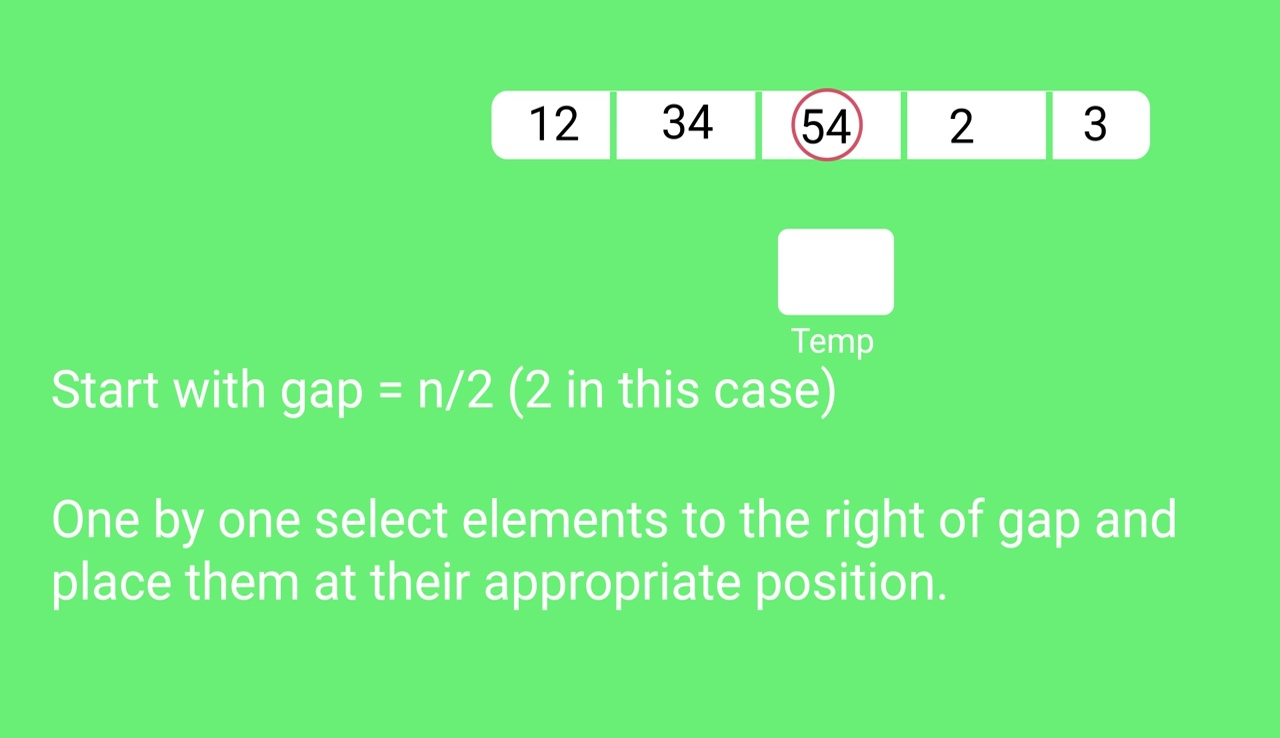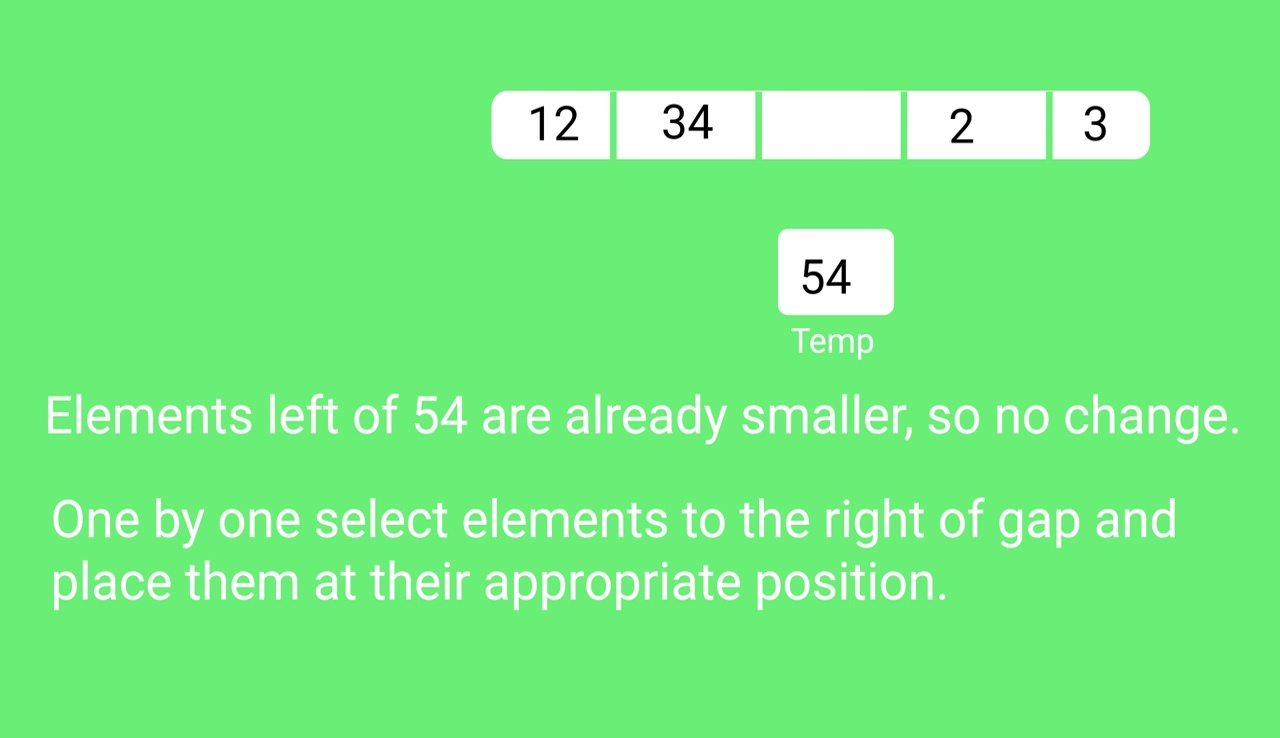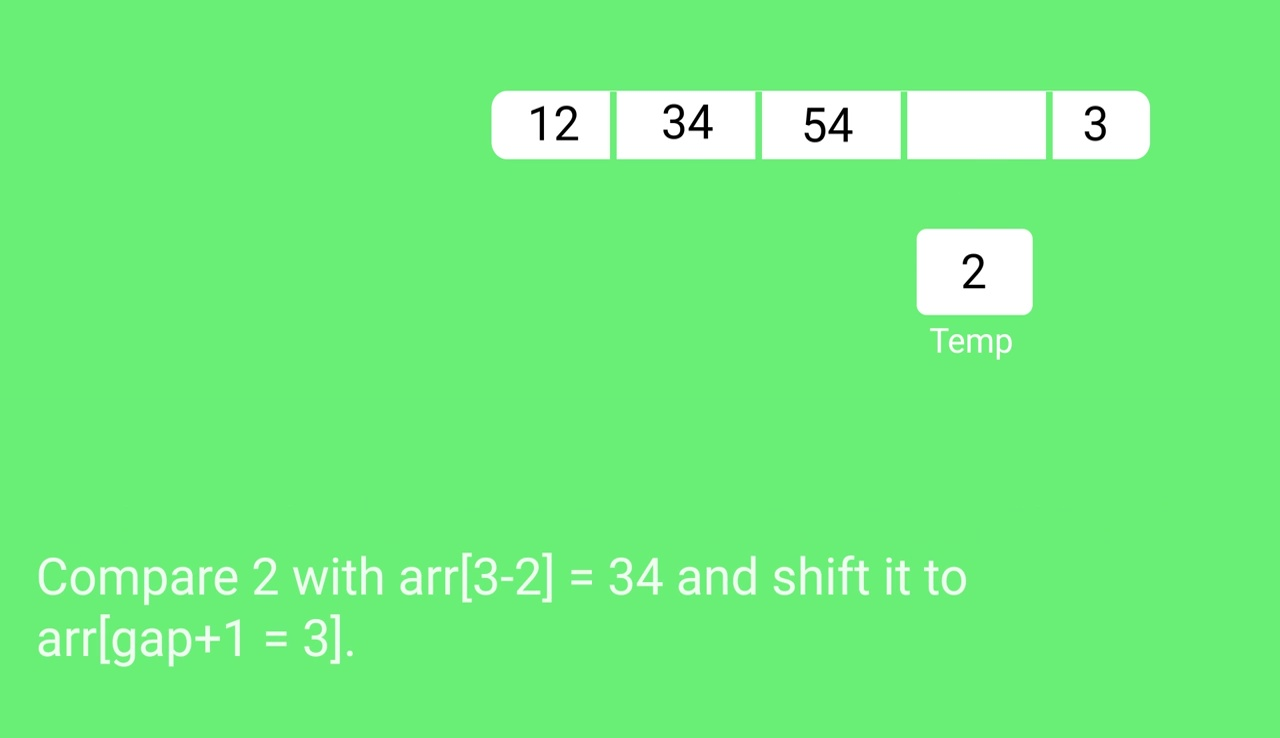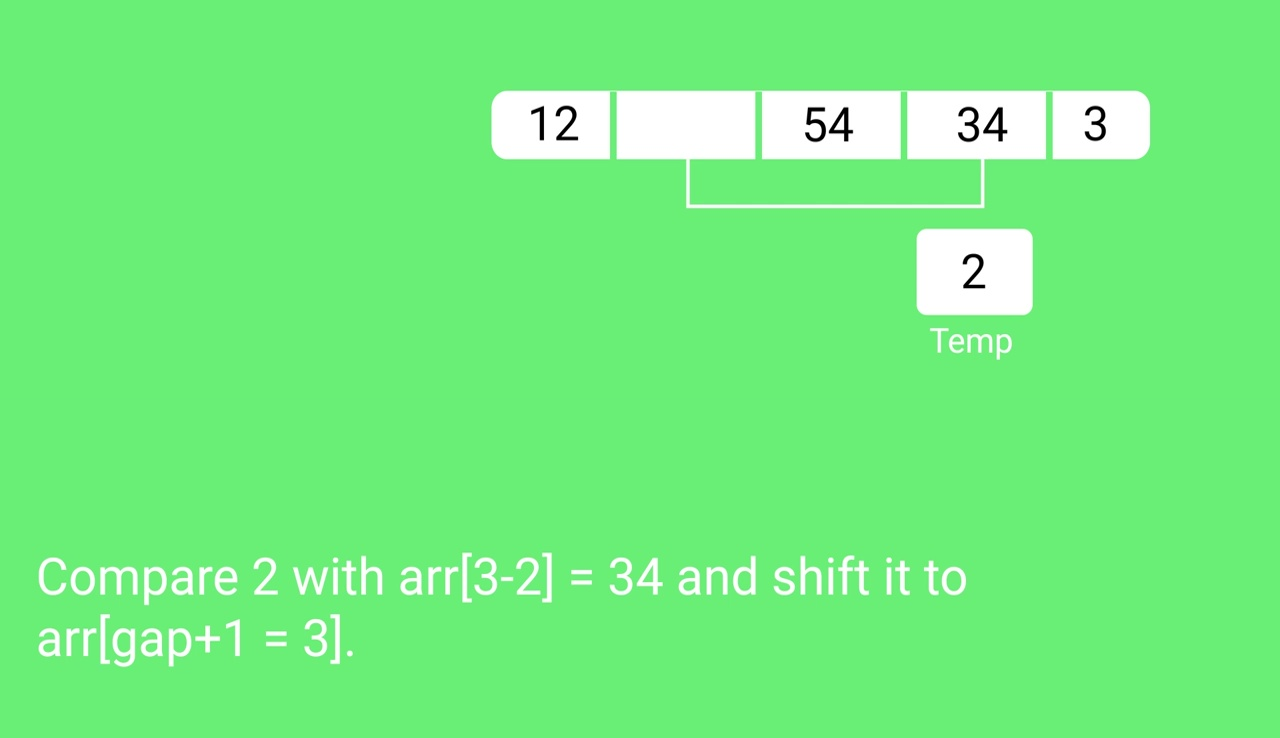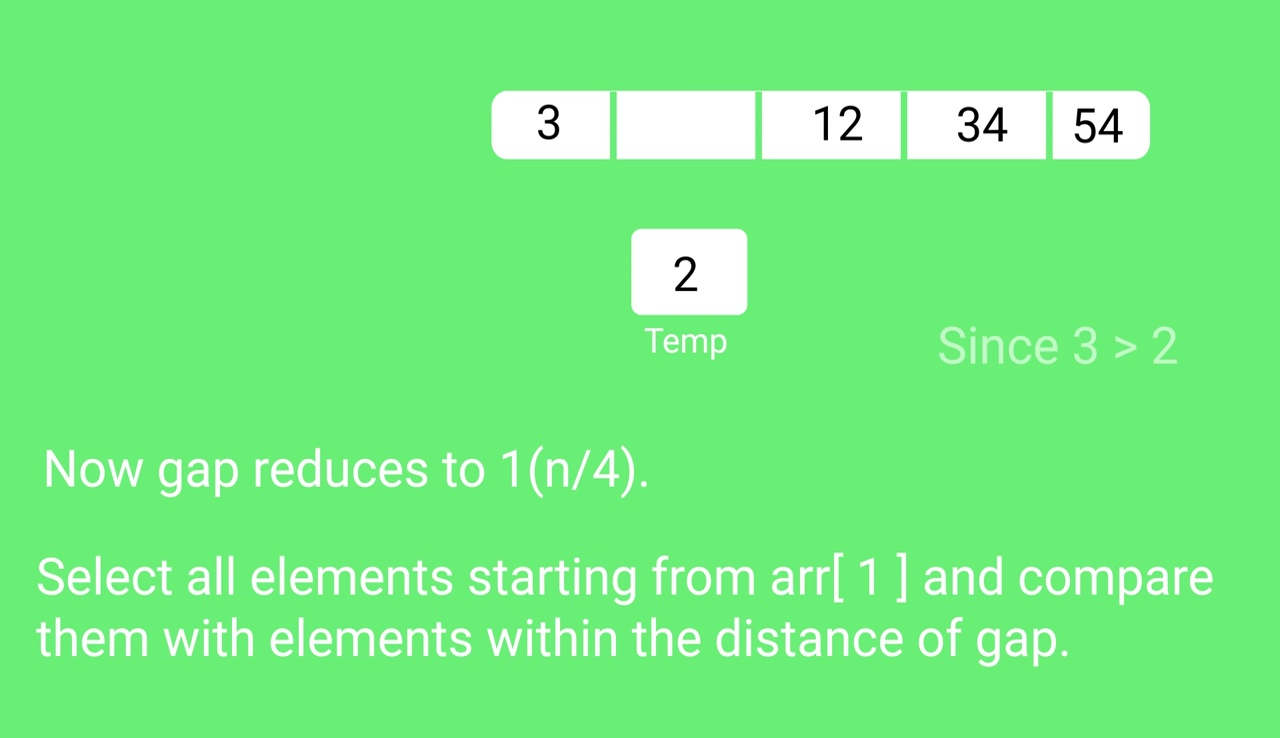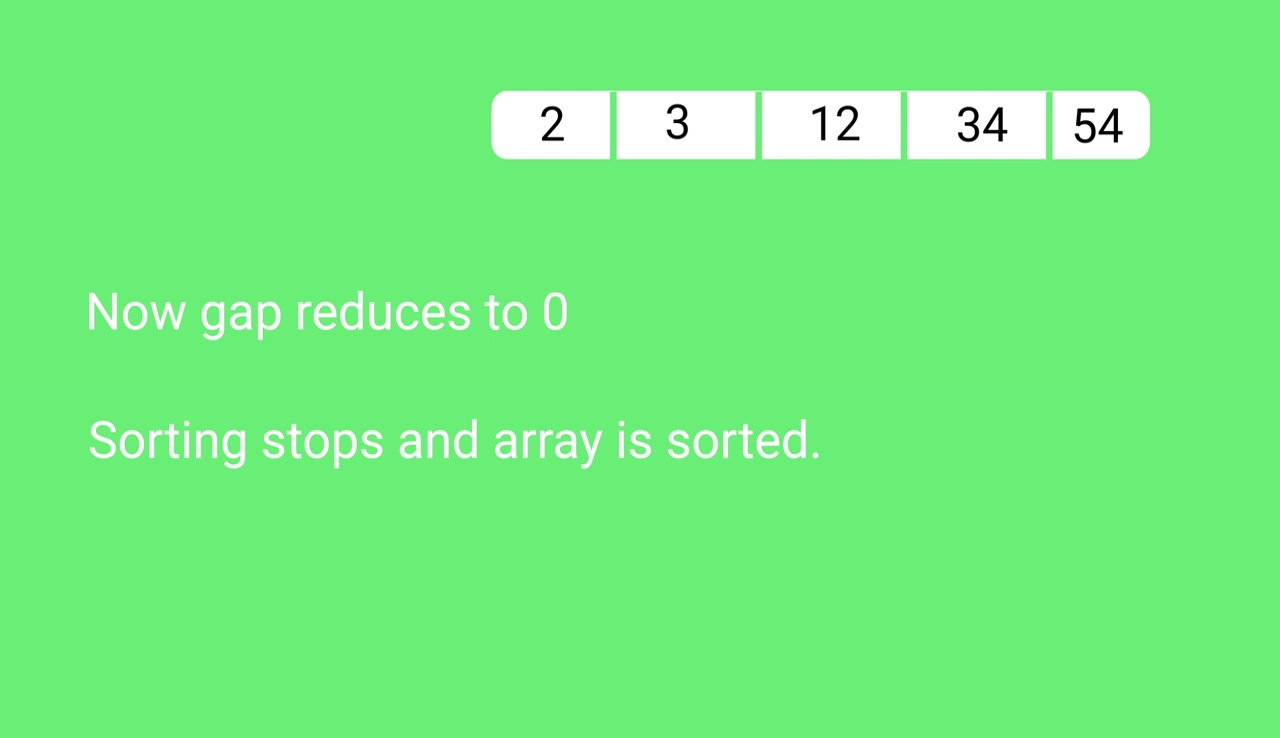## Quiz on Shell Sort

Other Sorting Algorithms on GeeksforGeeks/GeeksQuiz:

Feeling lost in the world of random DSA topics, wasting time without progress? It's time for a change! Join our DSA course, where we'll guide you on an exciting journey to master DSA efficiently and on schedule.
Ready to dive in? Explore our Free Demo Content and join our DSA course, trusted by over 100,000 geeks!

Previous
Next